## Nucleosynthesis: connecting nuclear physics to astrophysicsLA-UR-19-24624

### Matthew Mumpower

Frontiers Summer School

Thursday May 16$^{th}$ 2019Center for Theoretical
ASTROPHYSICS

## Outline

History lesson

Nucleosynthesis

Where nuclear physics comes in

Heavy element nucleosynthesis

Concluding remarks

## Observation: stars seem to shine for a long time...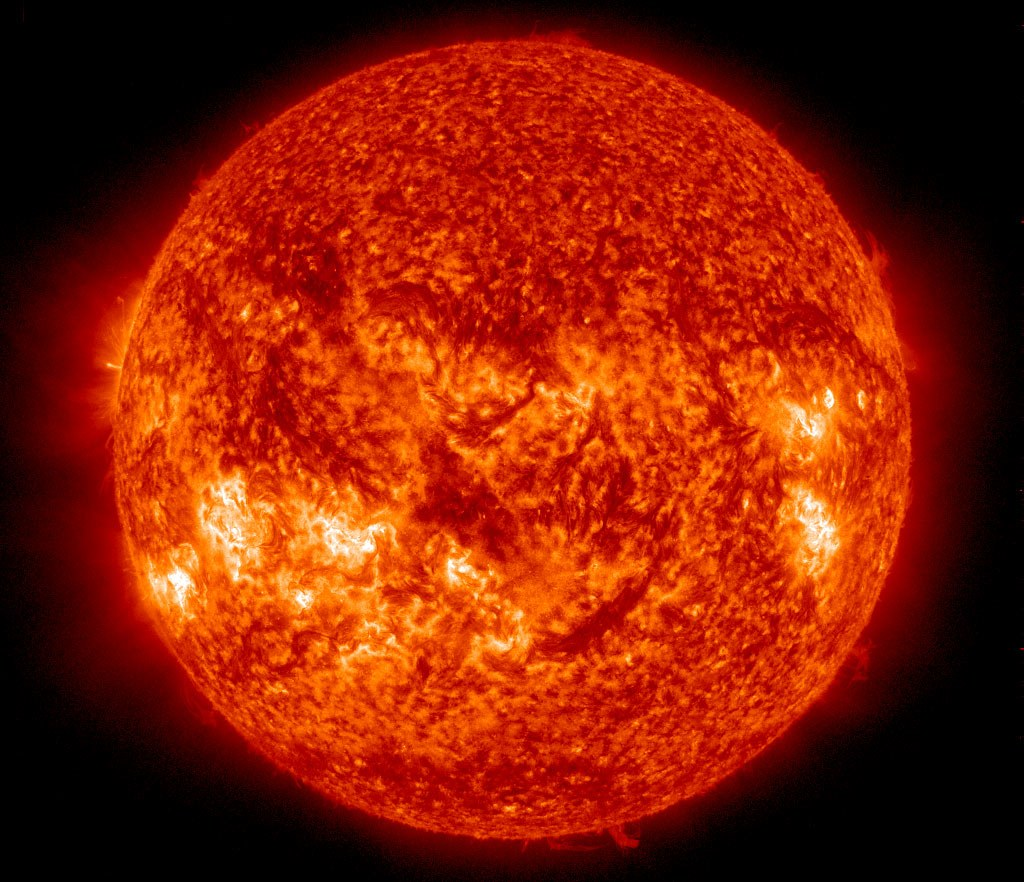How can we reconcile this observation with what we know?

## What could be powering stars?

Gravitational contraction: first proposed by Mayer, Helmholtz and lord Kelvin

Involves converting gravitational potential energy into heat

This was the leading postulate in the 1800's

Question: How long would the sun radiate under this assumption?

## lifetime $\sim$ (Fuel) / (Rate of Fuel use) = E / Q

Let's assume the heat from the sun is radiation dominated

Q = $\sigma$$T^{4}$$A$

$\sigma$: Stefan-Boltzmann constant

$T$: Temperature

$A$: Surface area

$Q \sim 5.67 \times 10^{-8} \times $${5800}^4$$ \times 6.09 \times 10^{12} \times 1000^{2} \sim $$3.9 \times 10^{26} Watts ## Gravitational energy? Considering gravitational potential energy ## E = \frac{3}{5}\frac{GM^{2}}{R} G: gravitational constant M: mass of the sun \sim 2 \times 10^{30} kg R: radius of the sun \sim 700 million meters E \sim 2.3 \times 10^{41} Joules So ... lifetime \sim (2.3 \times 10^{41}) / (3.9 \times 10^{26}) \sim 5.8 \times 10^{14} seconds 18 million years Nope... Who came up with the idea of using nuclear physics? ## Eddington's great insight Nuclear reactions release energy that power stars "The internal constitution of the stars" (1920) ## E = m c^{2} A little bit of mass can create a lot of energy Question: What percentage of mass will generate enough energy for the sun to last billions of years? (only 0.07% is needed) This was the start of nuclear physics + astrophysics → nuclear astrophysics Question: How long would the sun last if it ran on fossil fuels? ## Nucleosynthesis nu·cle·o·syn·the·sis The formation of new atomic nuclei by nuclear reactions, thought to occur in the interiors of stars and in the early stages of development of the universe.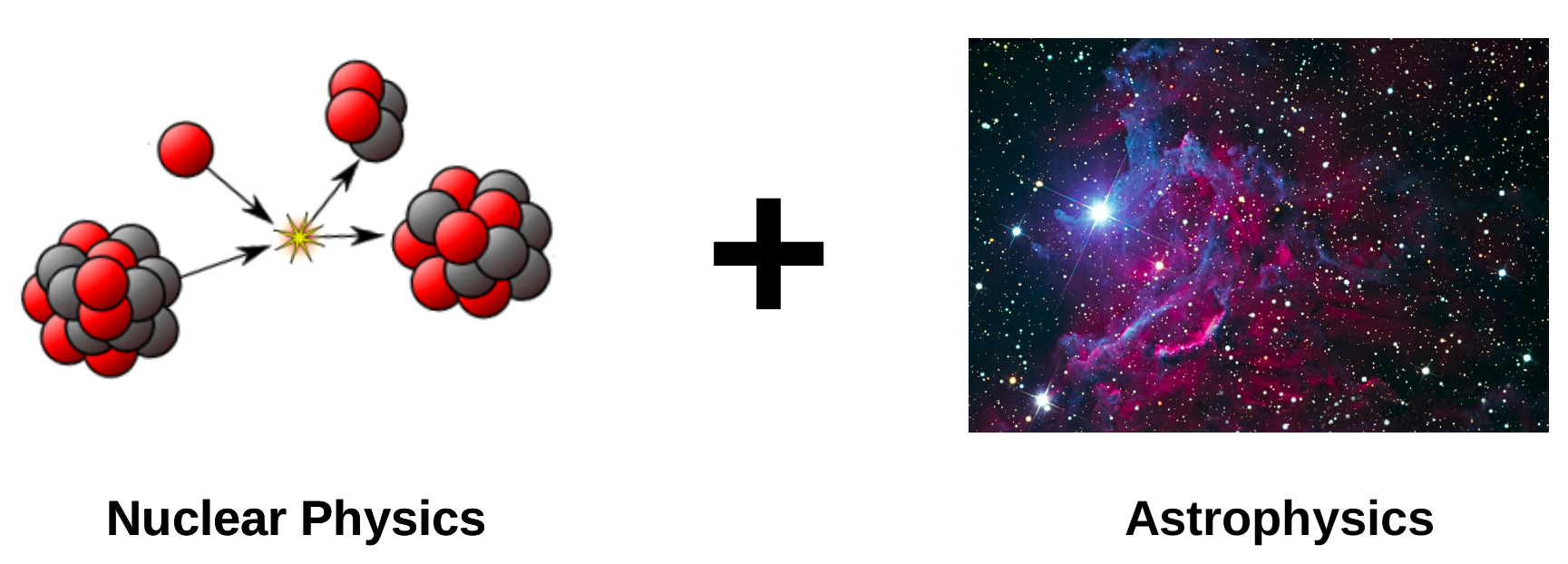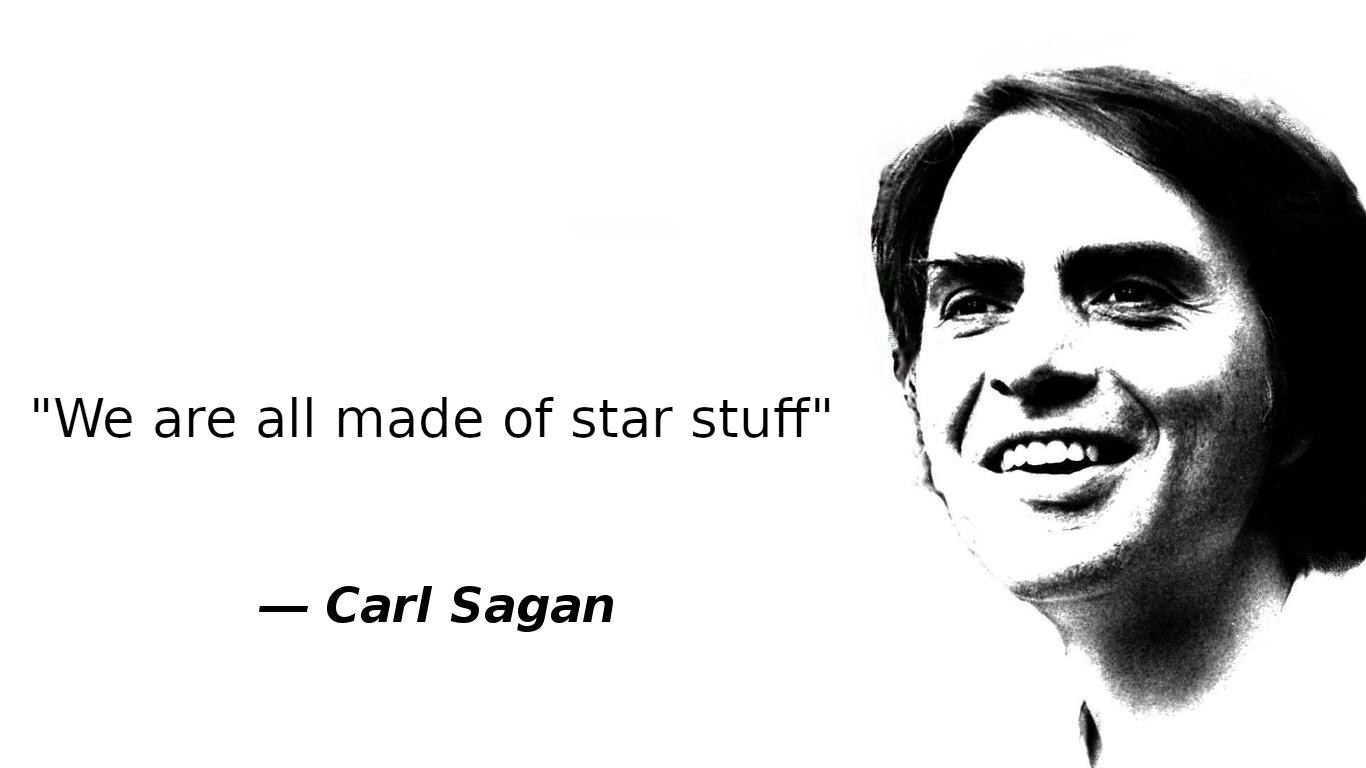## Stuff in the solar system Abundance is a quantity denoting how much stuff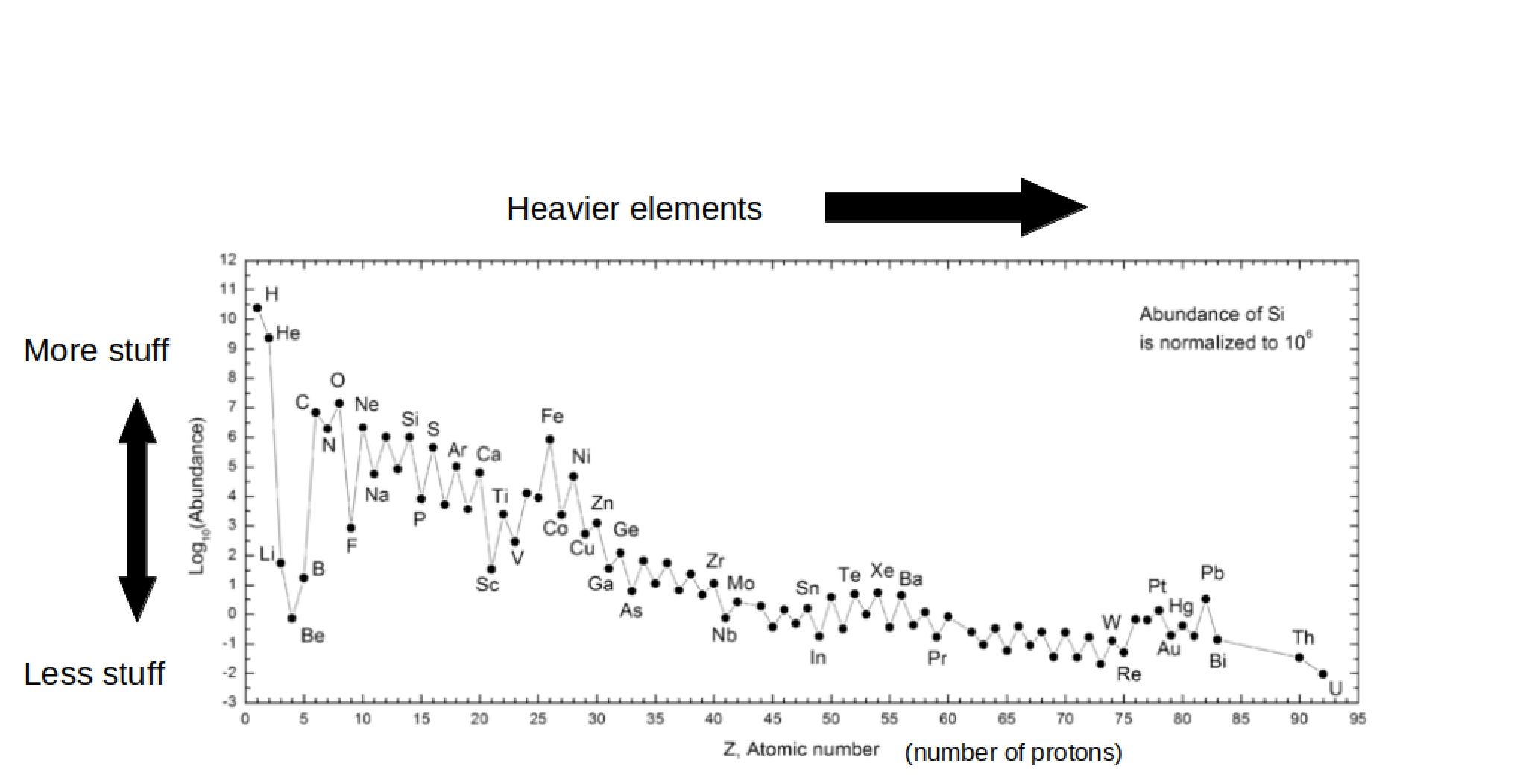Question: Where do we get this observational information? Hint: The sun has 99% of the mass of the solar system ## Stuff in the solar system (Answer: meteorites and photospheric observations)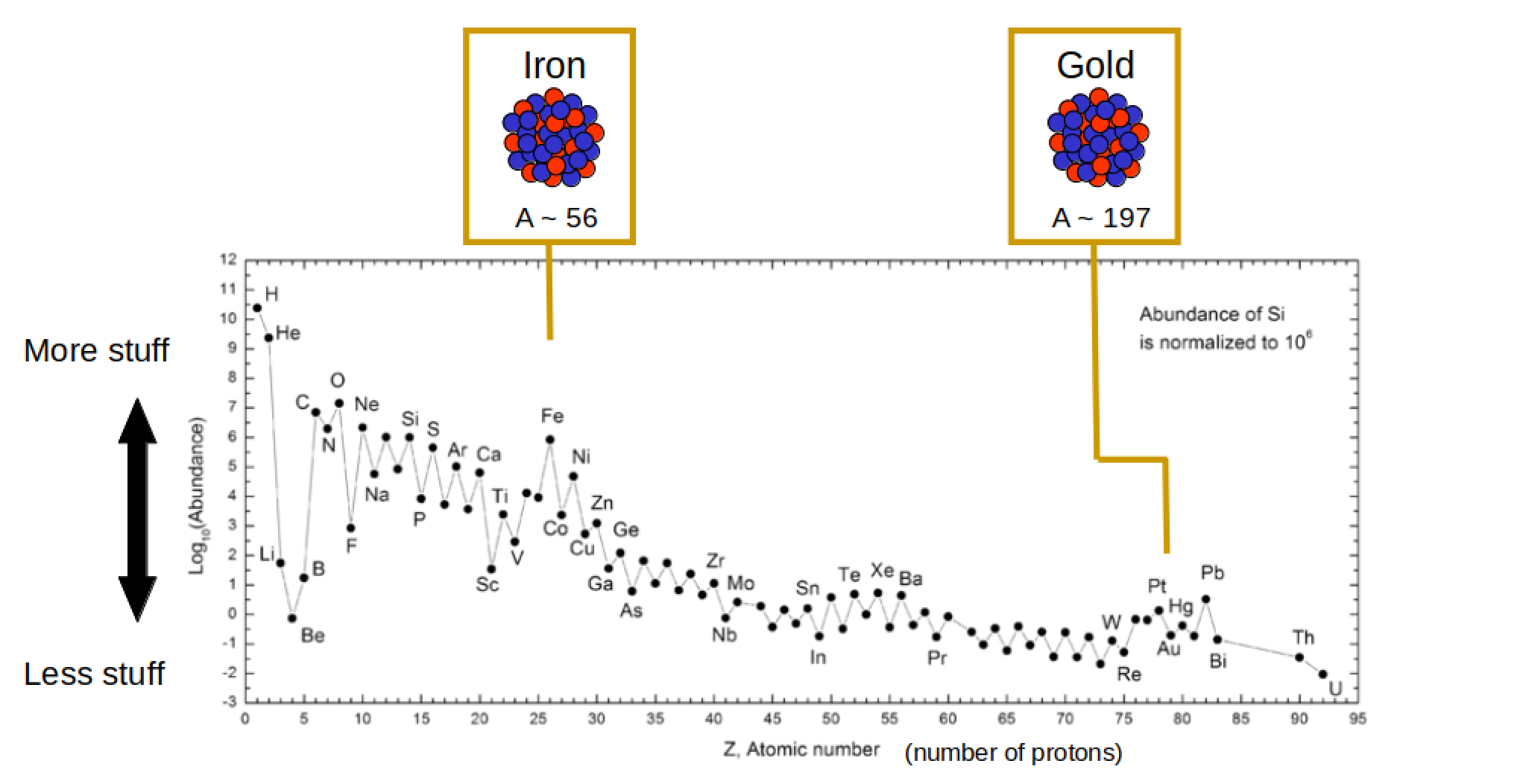The formation of the heavy elements didn't occur all at the same time nor the same place ## What is the origin of the elements?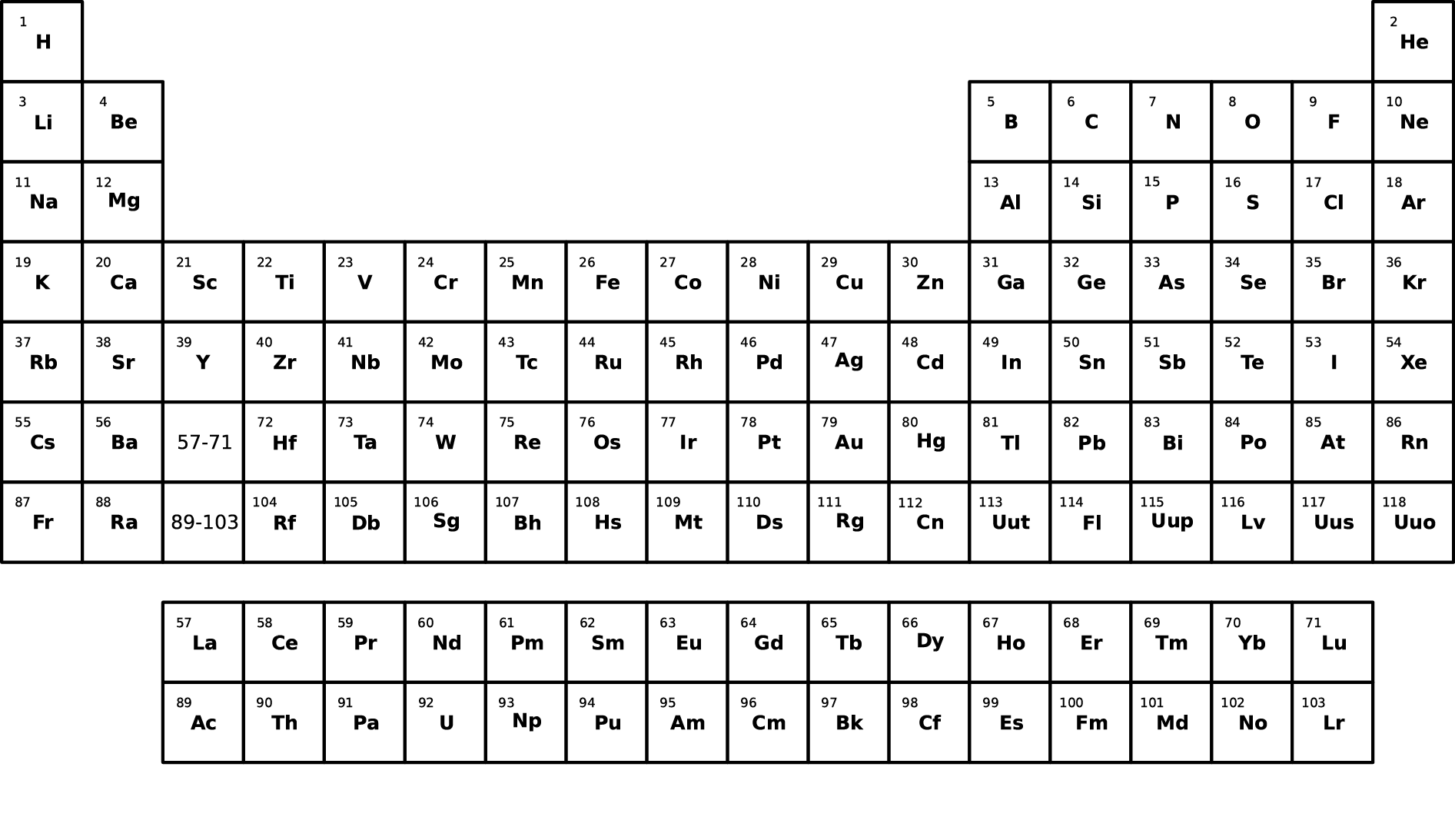This requires a lot of detective work... ## Lightest elements: big bang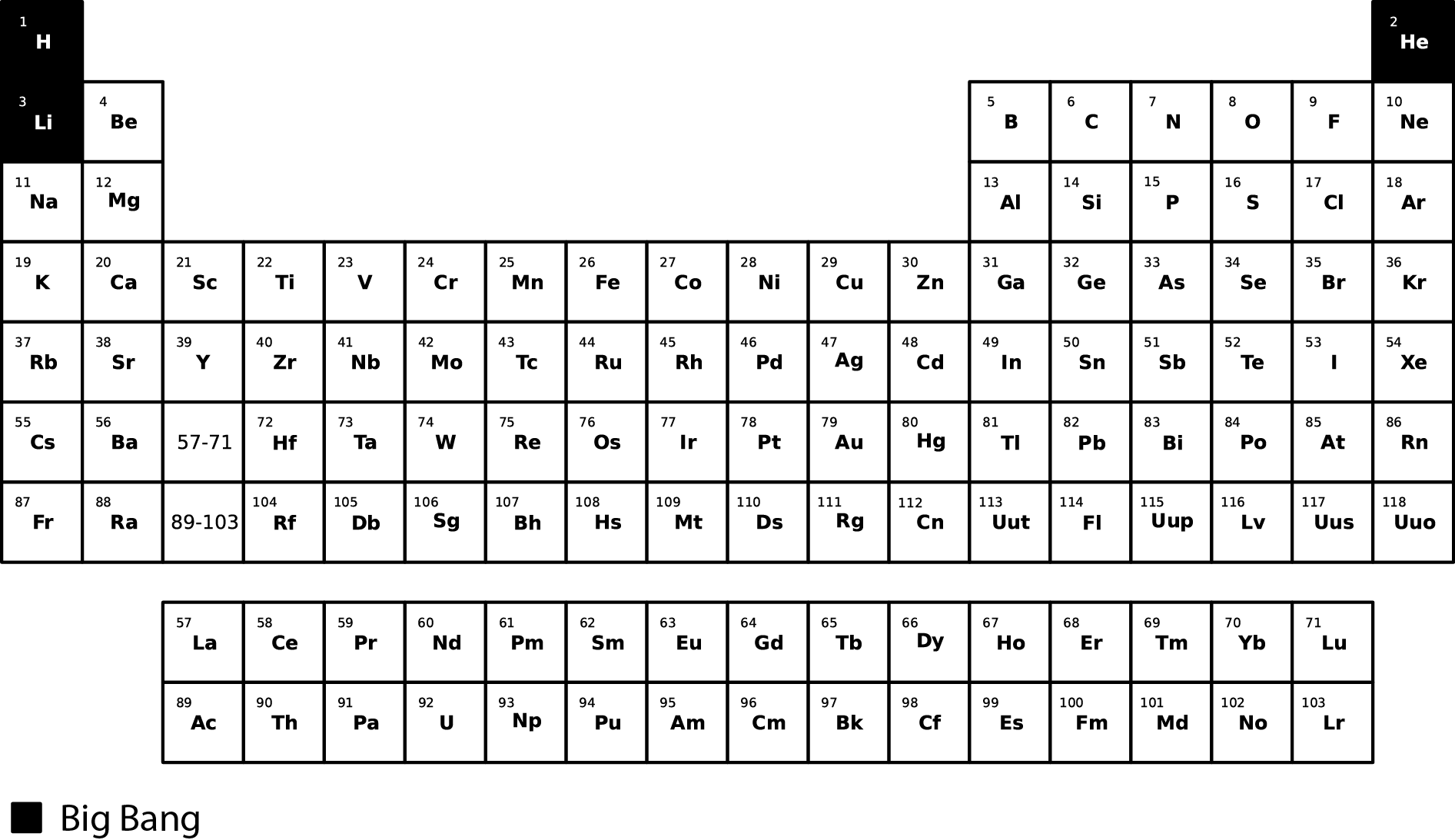## The Big Bang Created most of the hydrogen (H) and helium (He) in the universe. Started within the first 3 minutes of the beginning of the universe. Ended within about 20 minutes due to expanding and cooling. Only 12 key reactions to take into account (easy!?)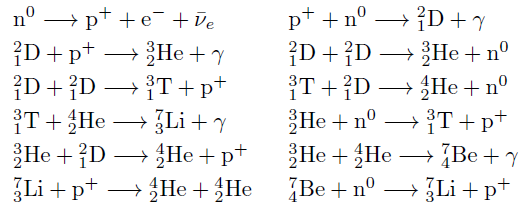Google: cosmic lithium problem ## Spallation by cosmic rays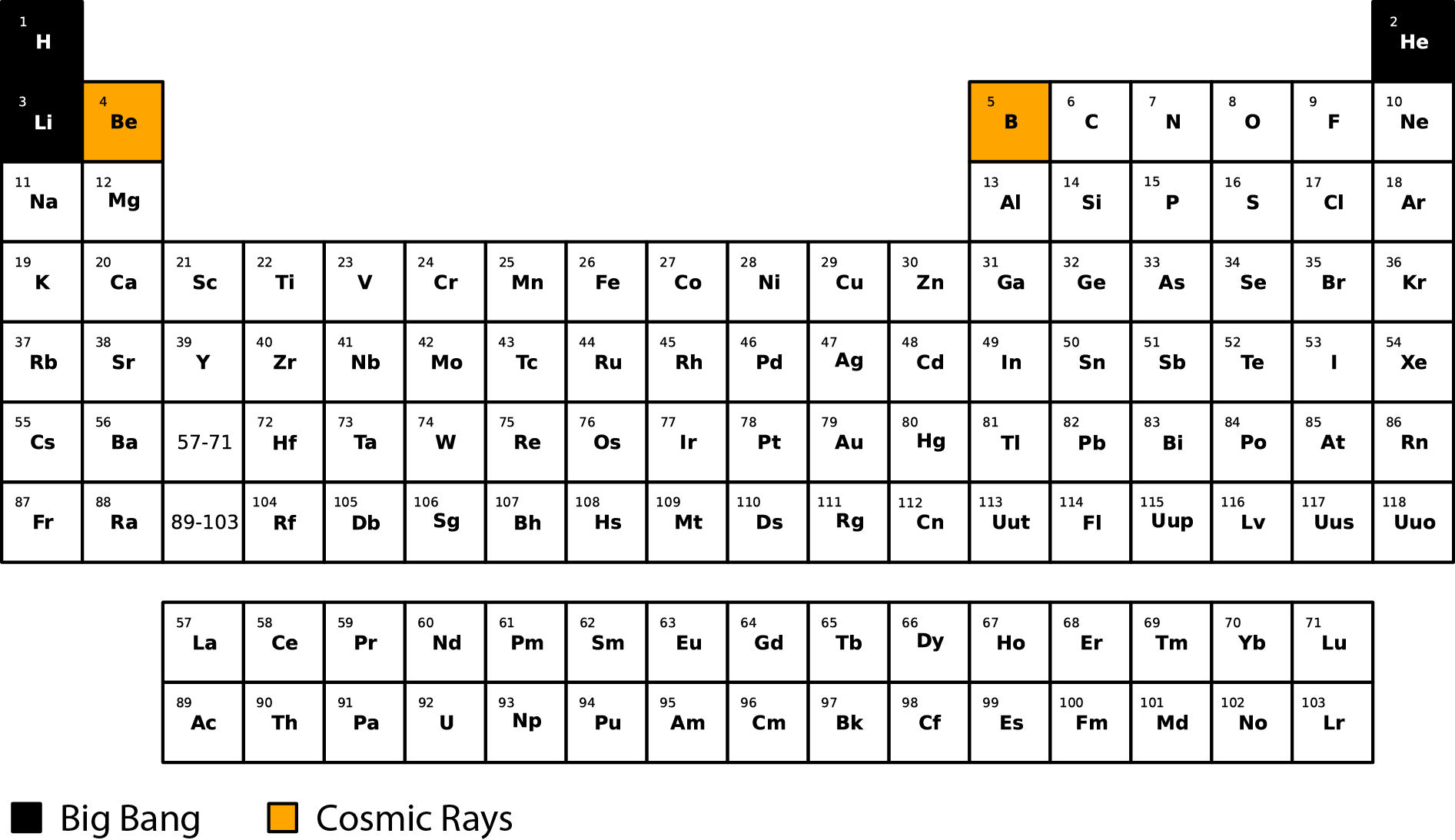Remember the dip in the solar abundances? ## Stellar burning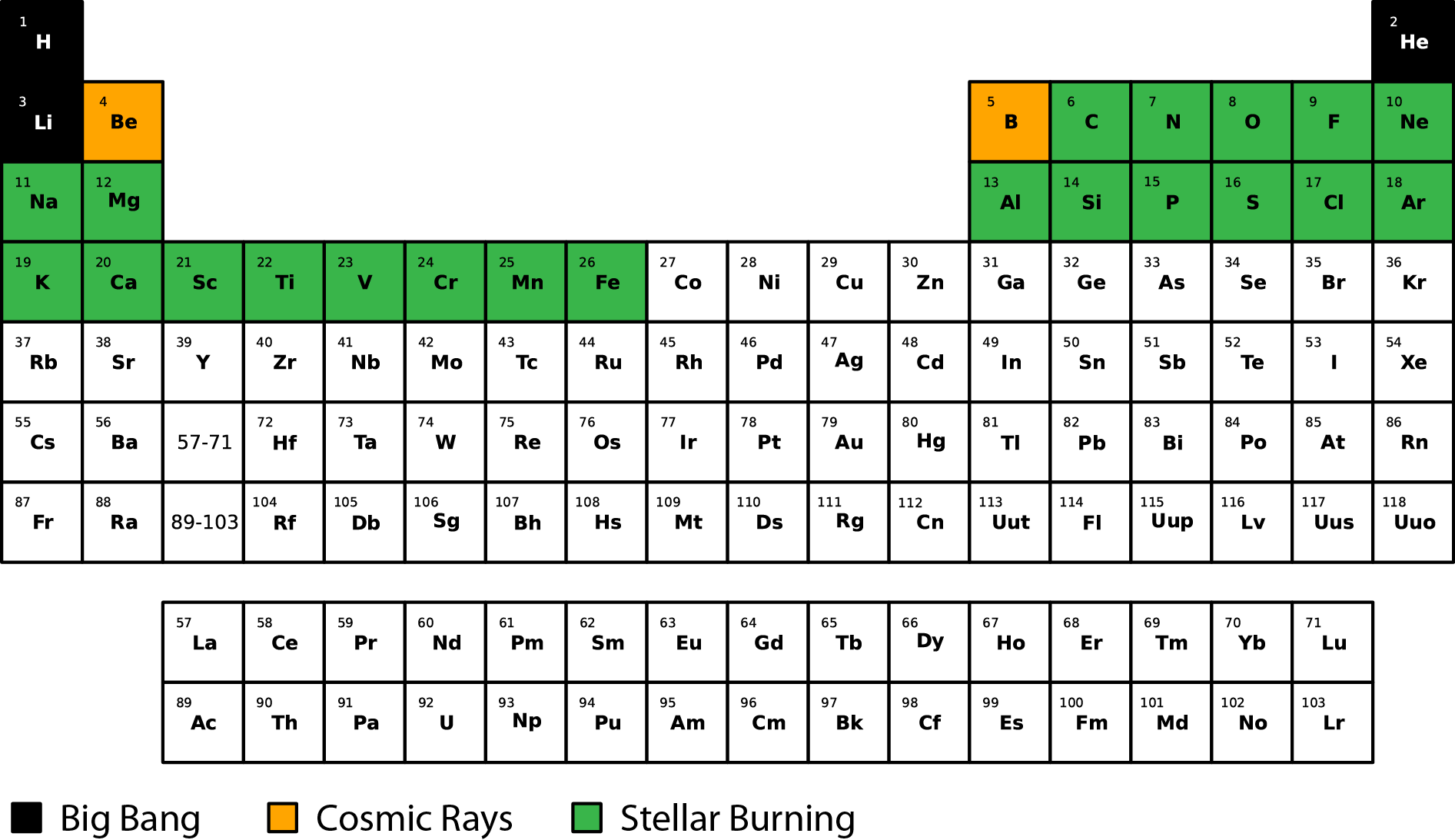Recall Jinmi Yoon's talk ## Stellar burning Nuclear fuel for the existence of stars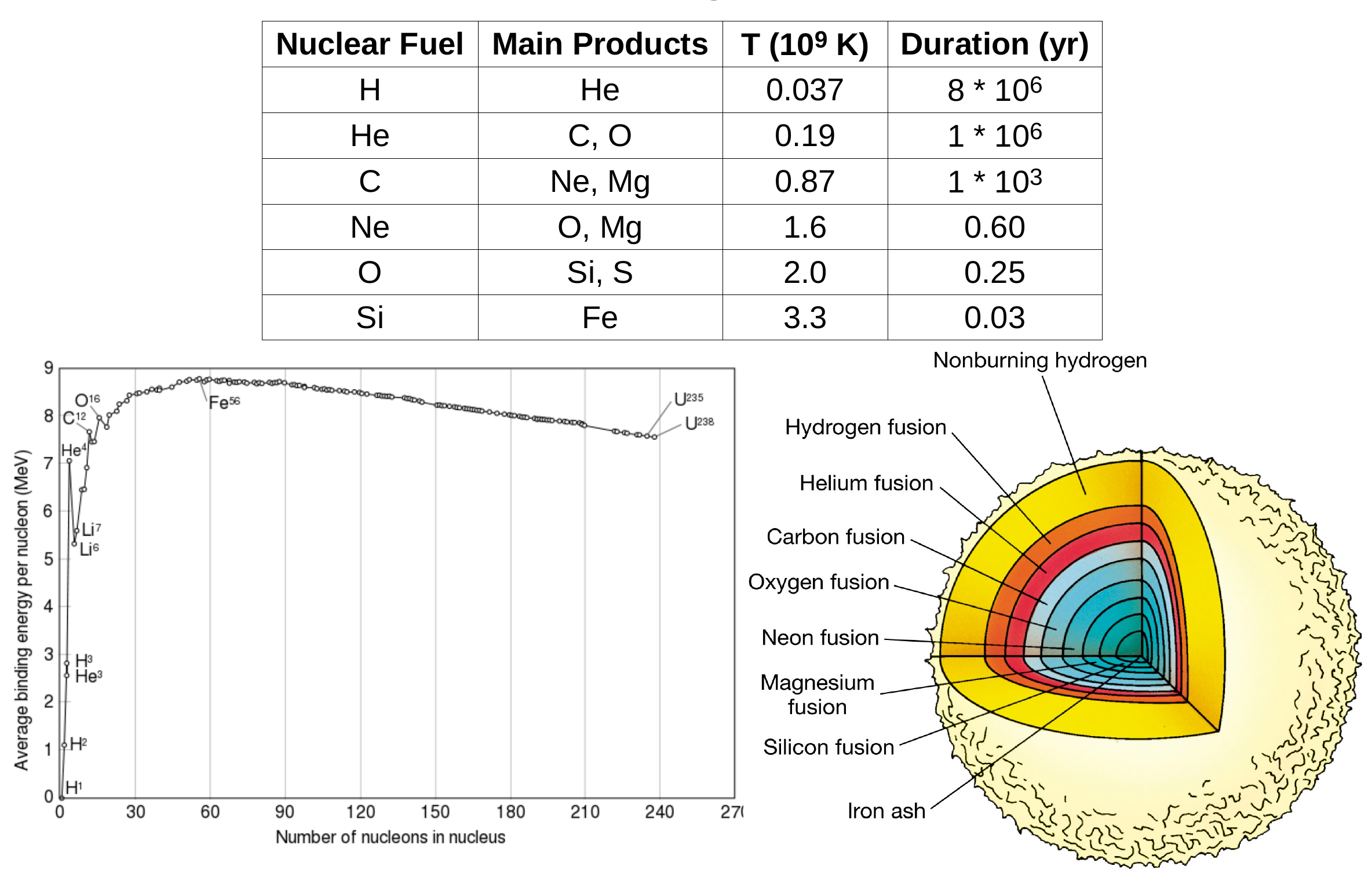Google: onion model of stars ## Heavy elements: dying stars, compact objects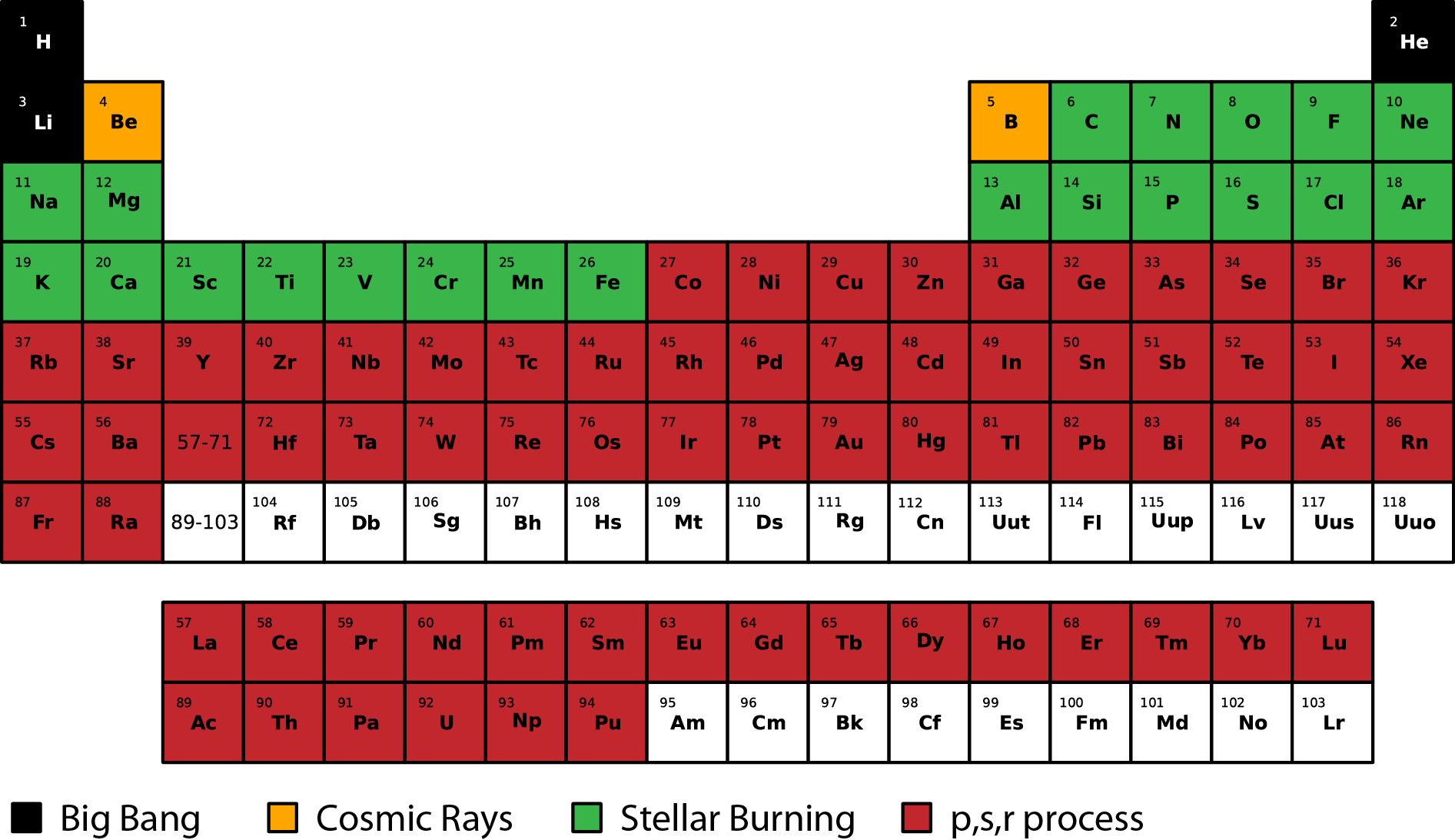This is area is a hot topic of current research... ## Heaviest elements: man made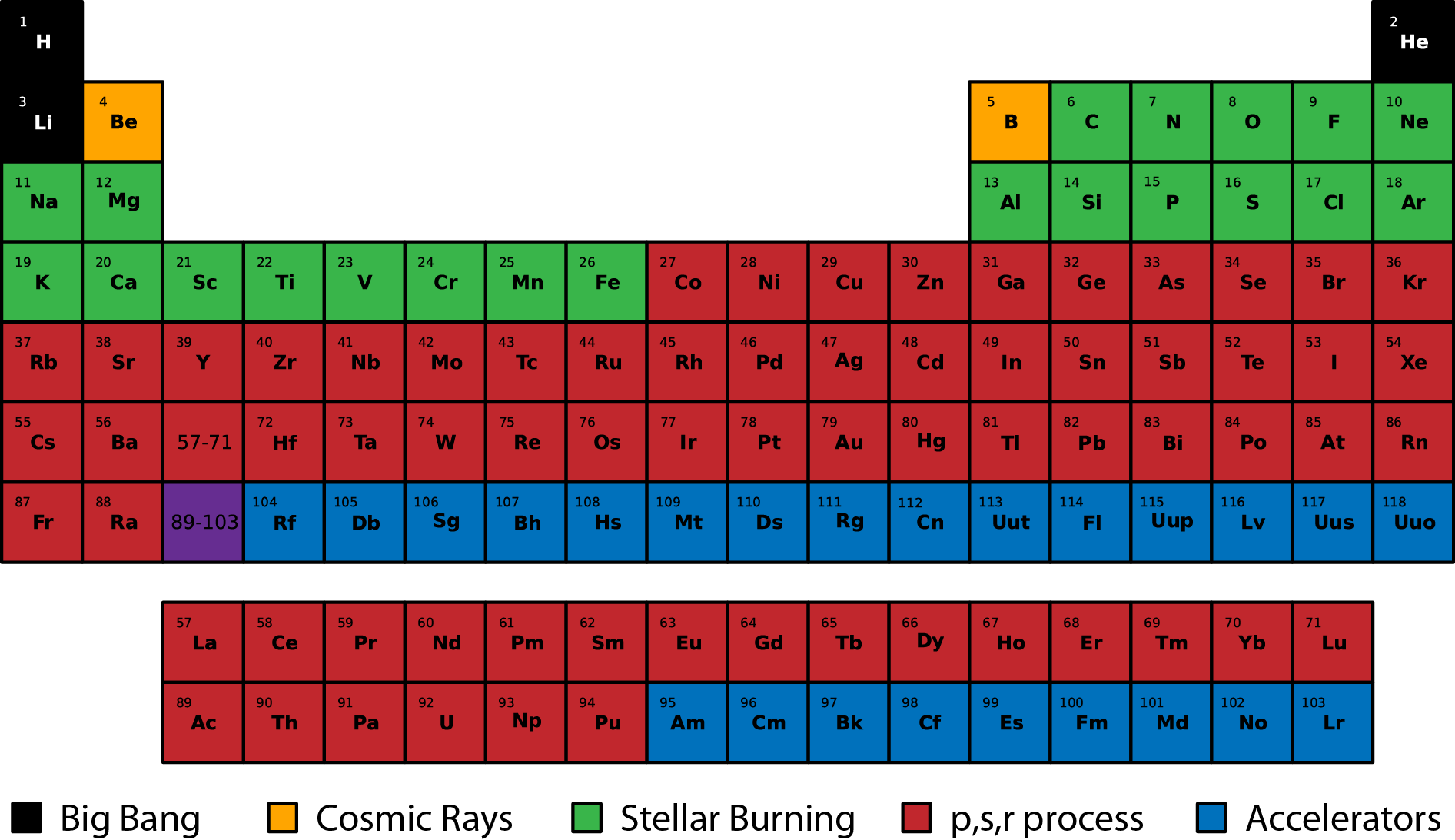Google: what is the heaviest man made element? ## Further evidence of nuclear physics in astrophysics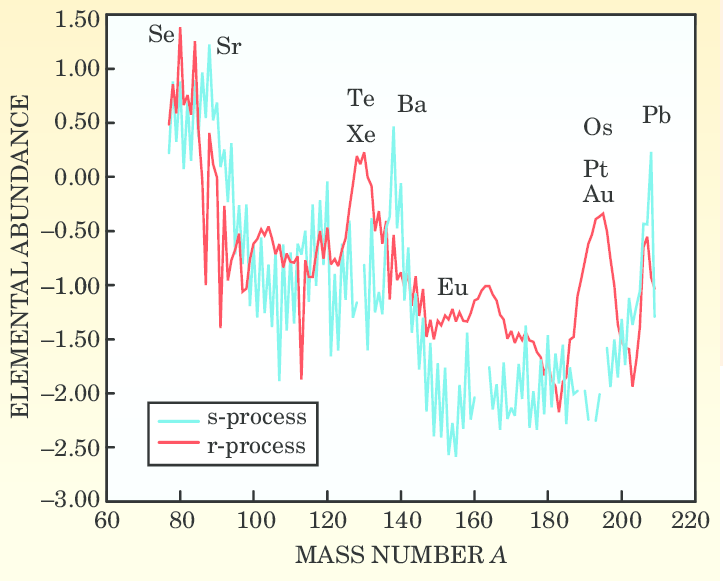In the isotopic abundances there were two bumps This implies two different processes are happening Google: Suess & Urey abundance curve ## Further evidence of nuclear physics in astrophysicsQuestion: What is causing the major bumps (peaks)? Nuclear structure! Google: BBFH (Burbidge Burbidge Fowler and Hoyle) ## The slow neutron capture process s-process: neutron capture rates are slow relative to \beta-decay; \tau_n$$ \gg $$\tau_\beta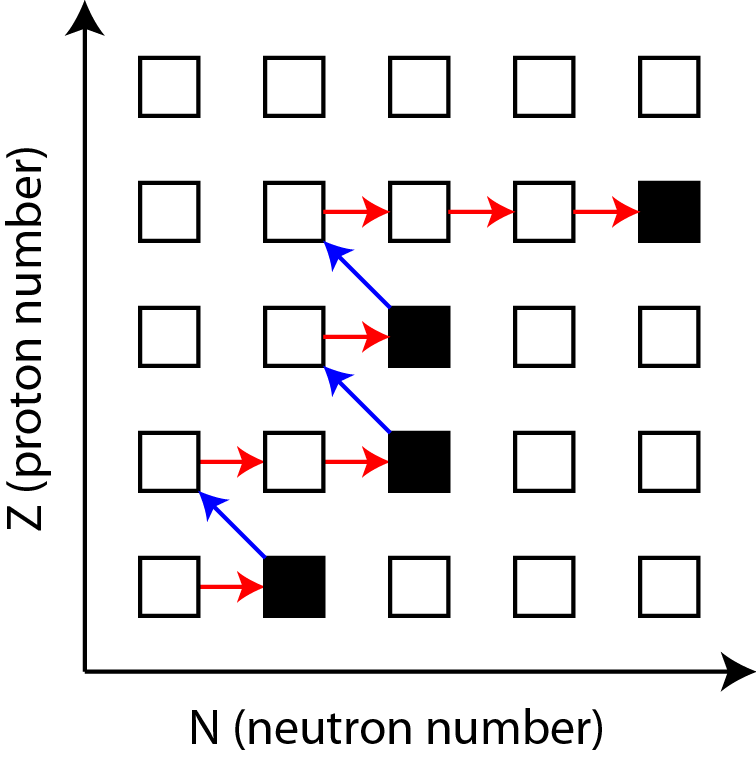(Z,N) + n ↔ (Z,N+1) + \gamma (Z,N) → (Z+1,N-1) + e^{-} + \bar{\nu}_{e} ## How do we form the peaks? s-process: neutron capture rates are slow relative to \beta-decay; \tau_n$$ \gg $$\tau_\betaThis process stays very close to the stable isotopes • most nuclear physics inputs are known Primarily occurring in AGB stars ## Radiative Neutron Capture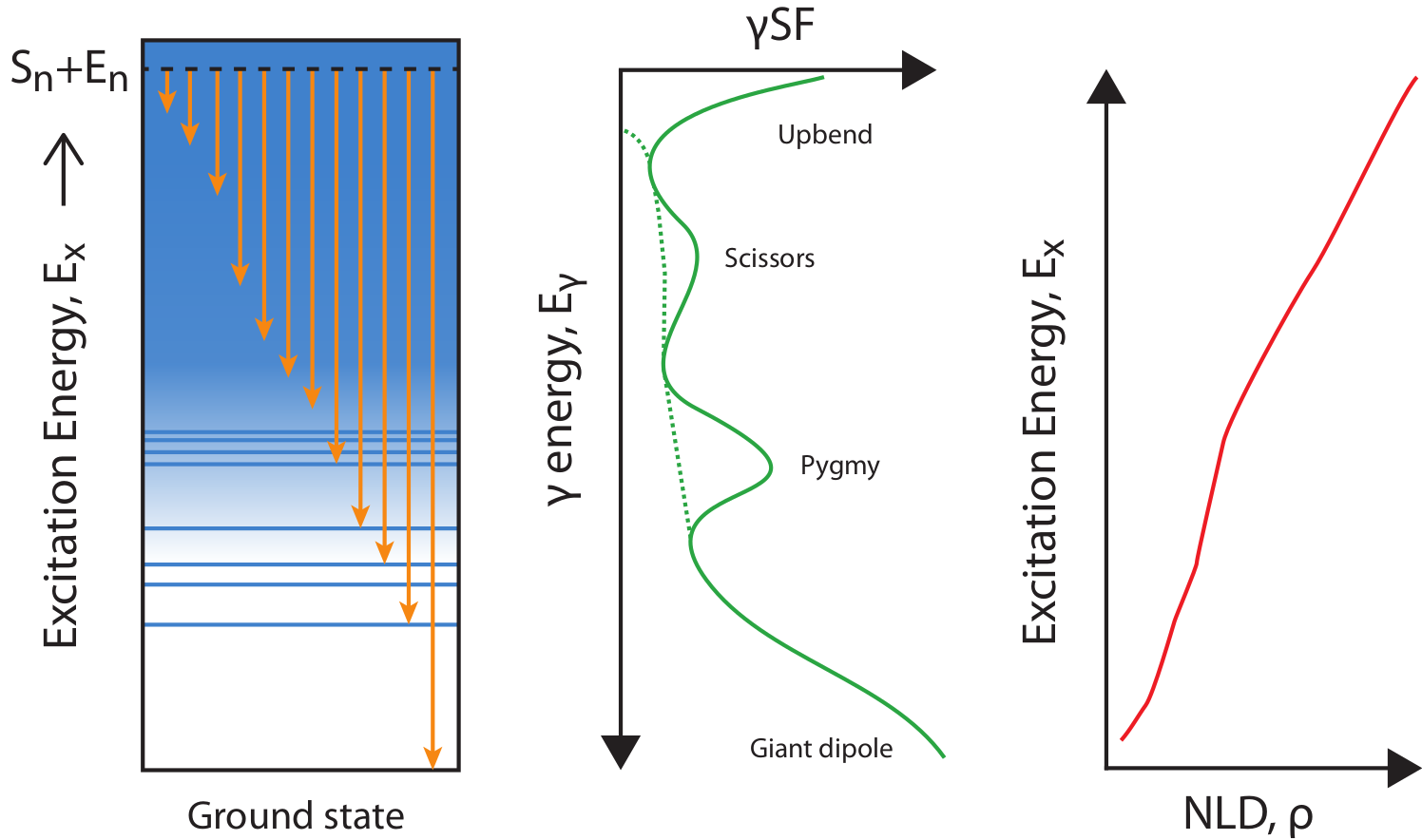(Z,N) + n ↔ (Z,N+1) + \gamma Key components: Optical potential\gamma-ray strength function (\gammaSF)Nuclear Level Density (NLD) Google: R-matrix theory • Hauser-Feshbach theory ## Nuclear \beta^{-}-decay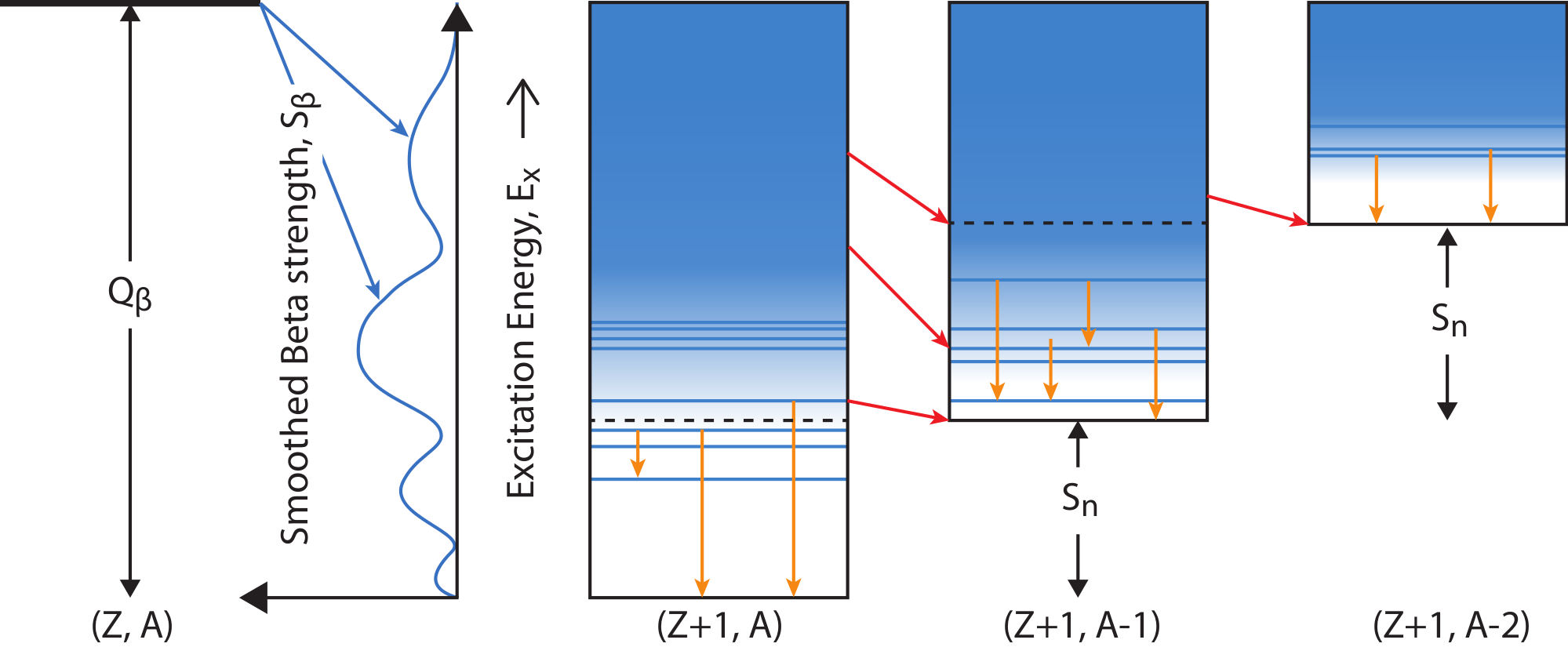(Z,N) → (Z+1,N-1) + e^{-} + \bar{\nu}_{e} Key components: Fermi's Golden Rule • nuclear levels • binding energies • \gammaSF / NLD As we add neutrons: Q_{beta} ⇡ S_{n} ⇣ so what happens? We release more neutrons! Google: Quasi-particle Random Phase Approximation (QRPA) ## What happens when \tau_n$$ \ll$$\tau_\beta$?We're going to go far from the stable isotopes (further to the right)!

This is known as the rapid neutron capture process ($r$-process)

## The $r$-process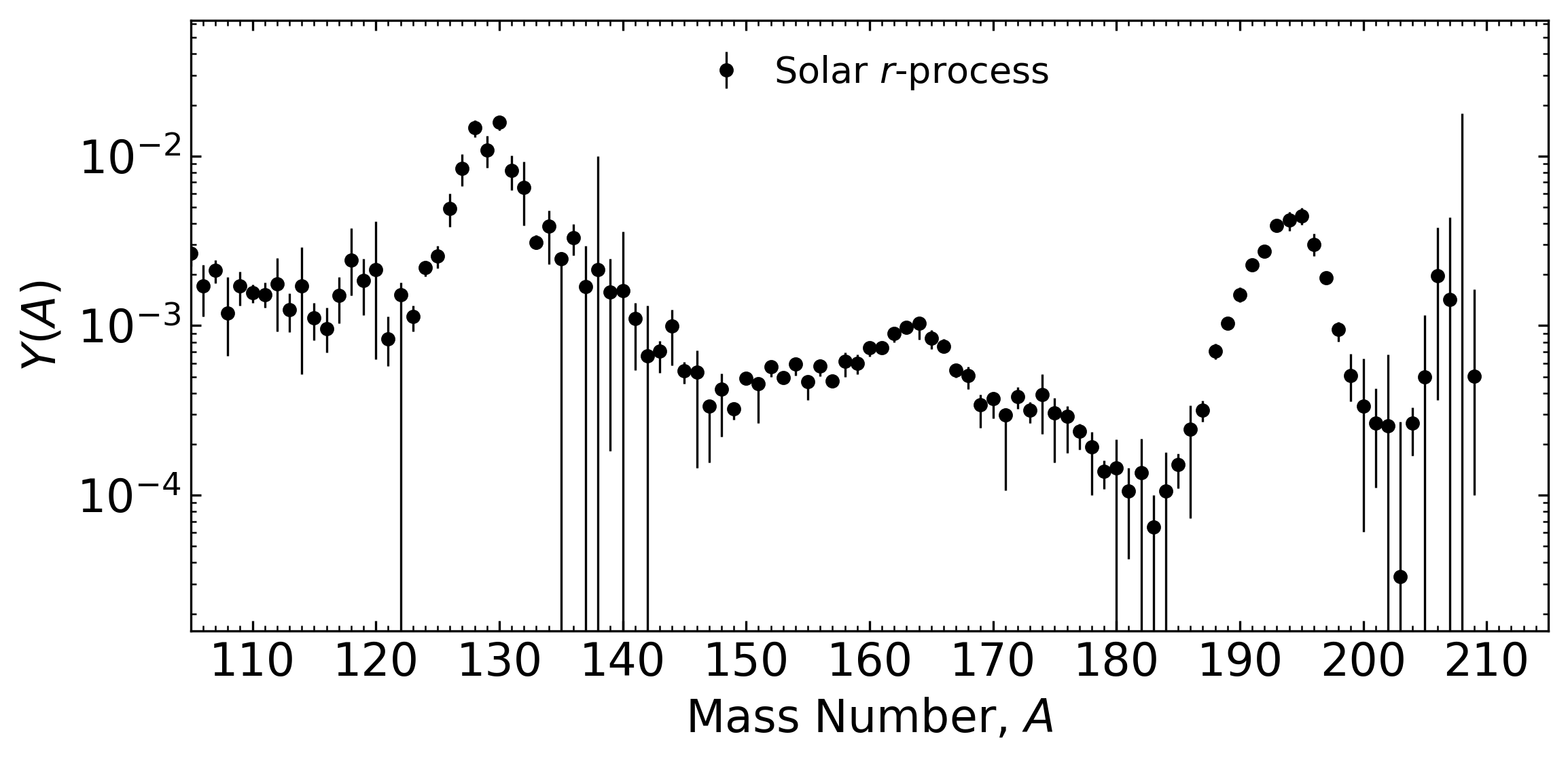Believed to be responsible for roughly half the elements above iron

All of the actinides are produced by this nucleosynthesis process; many neutrons required

Major problem: We only have hints of where this process occurs in nature

Another major problem: we barely have any nuclear data in this region

Why?... nuclei are short-lived

## One possible candidate site: supernova

End of the life of a massive star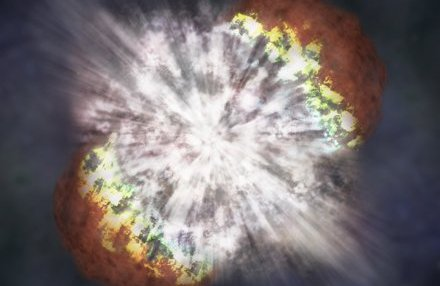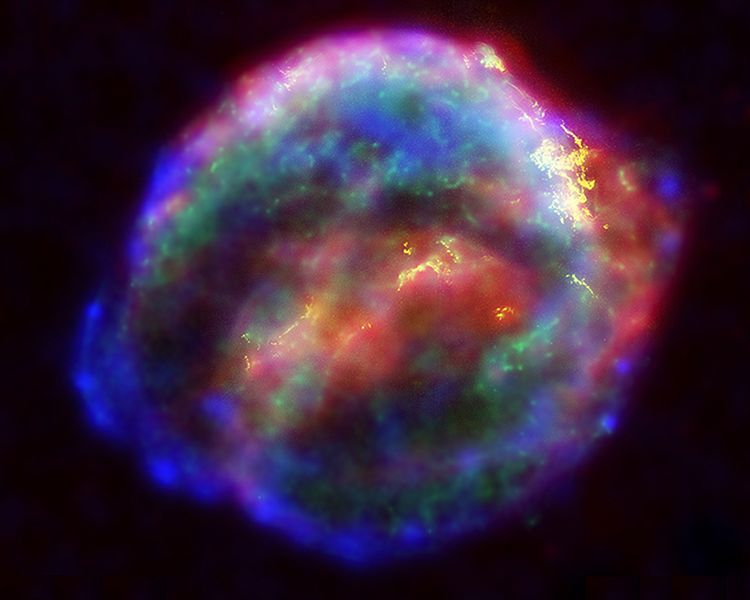Extremely luminous - burst of radiation that can outshine host galaxy for several weeks expelling the star's material

Can it produce neutron-rich material? This is under debate... MHD jets?

Requires exascale computing to properly model in full 3D

## Another candidate site: compact object mergers

Merger of two neutron stars • merger of neutron star with black hole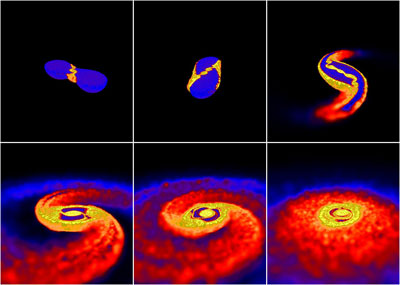Very rare events • lots of neutrons! • different types of ejecta

## Nuclear physics difficulties of the $r$-process

Every possible neutron-rich species that could exist in nature may be accessed (1000's)

Problem: we have some (incomplete) data for several hundred...

We need binding energies, decay rates, branching ratios, reaction rates, even fission information

There's no way around this... we require nuclear theory

## Nuclear physics as the language of the $r$-process

1st order: masses, $\beta$-decay rates, capture rates & fission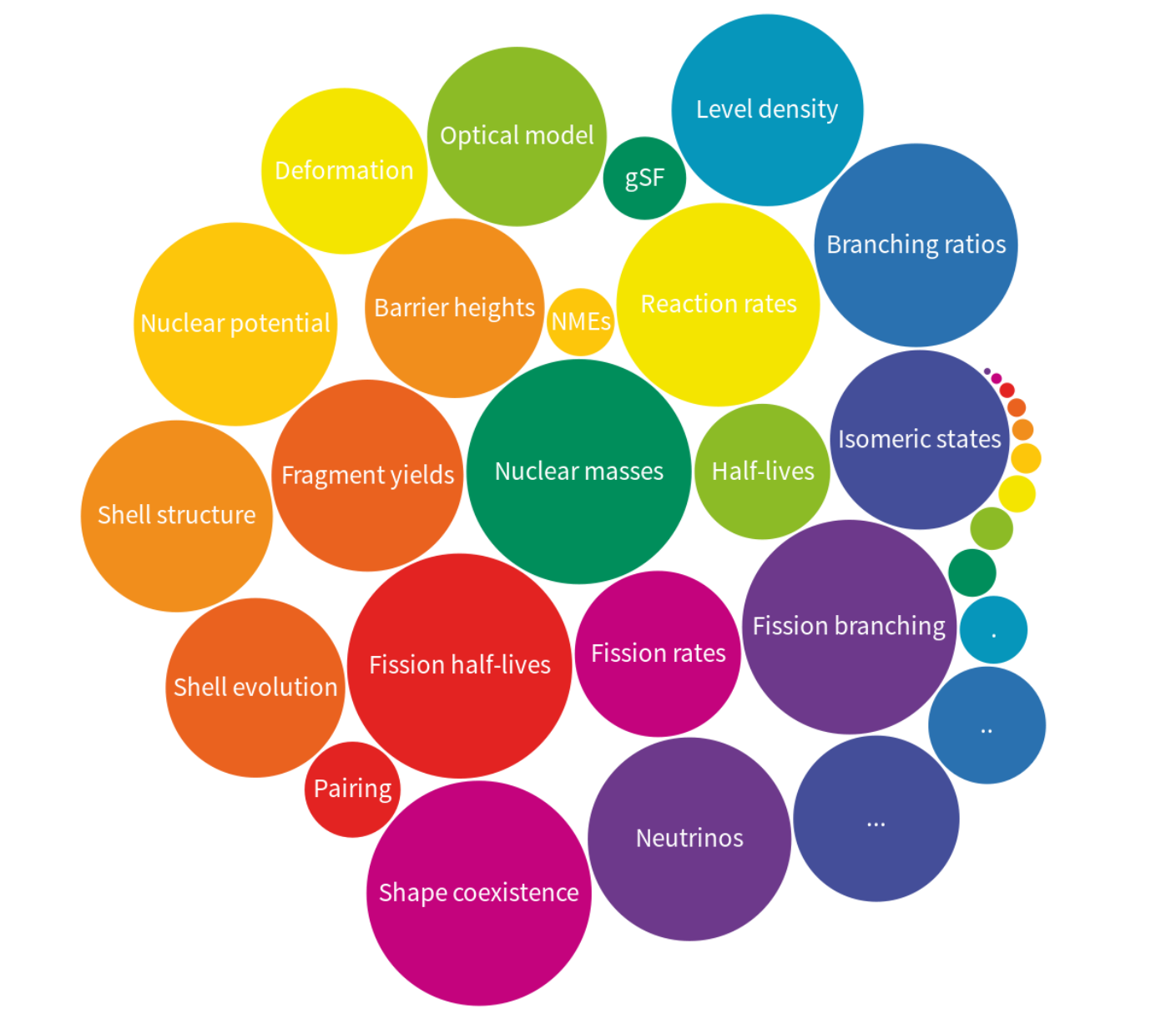## What do we know?

The chart of nuclides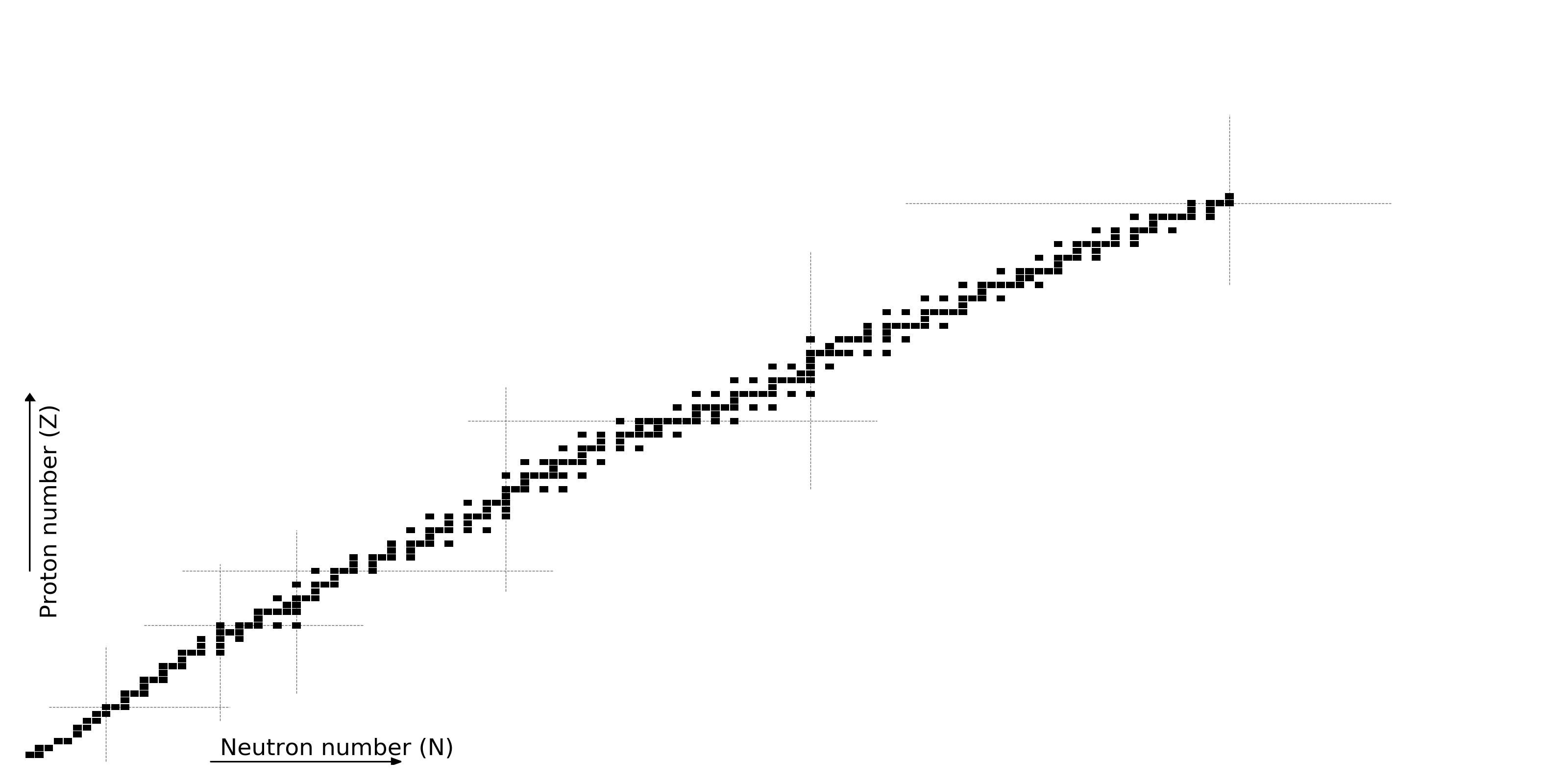## What do we know?

All half-lives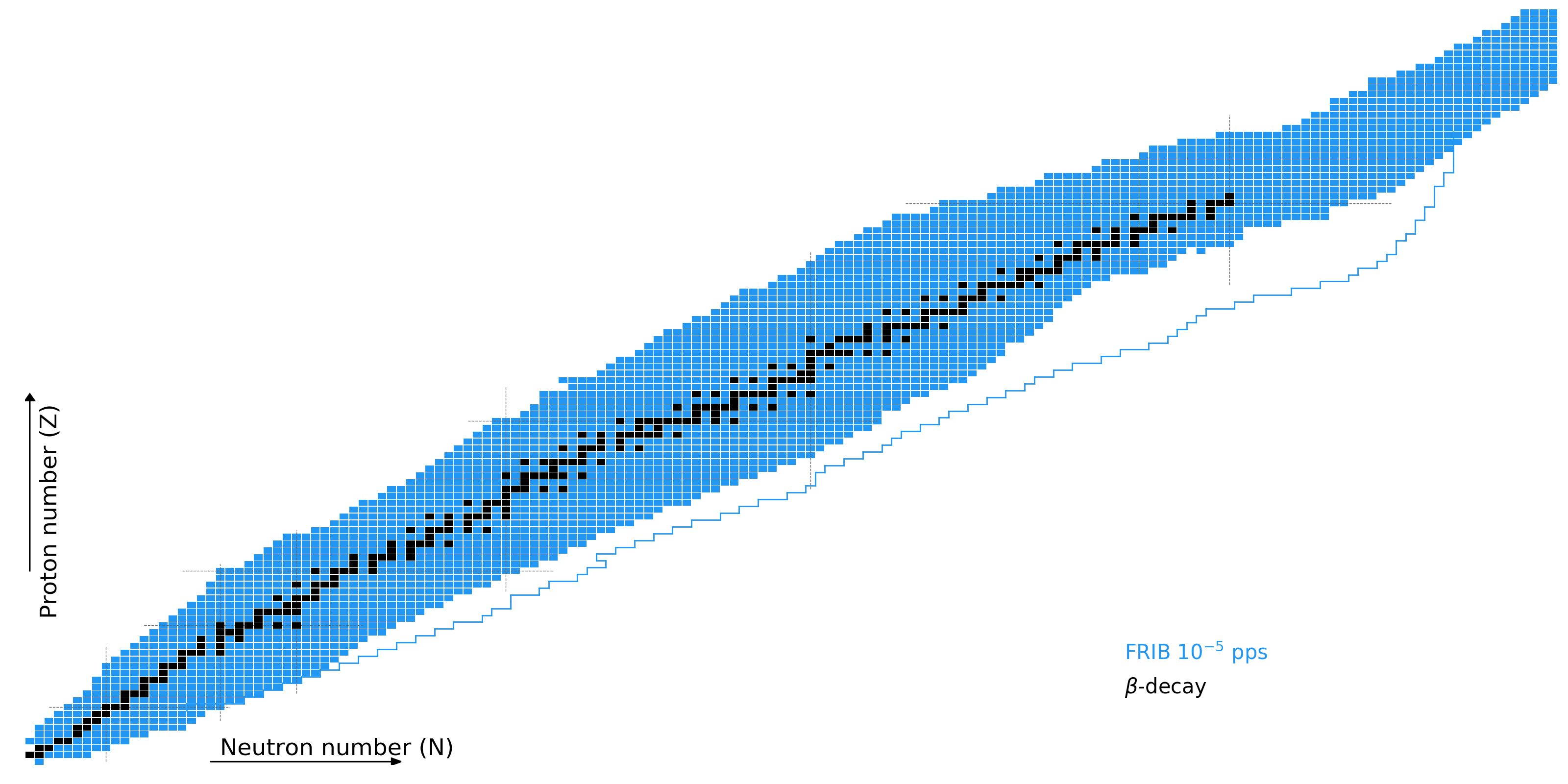## What do we know?

Nuclear masses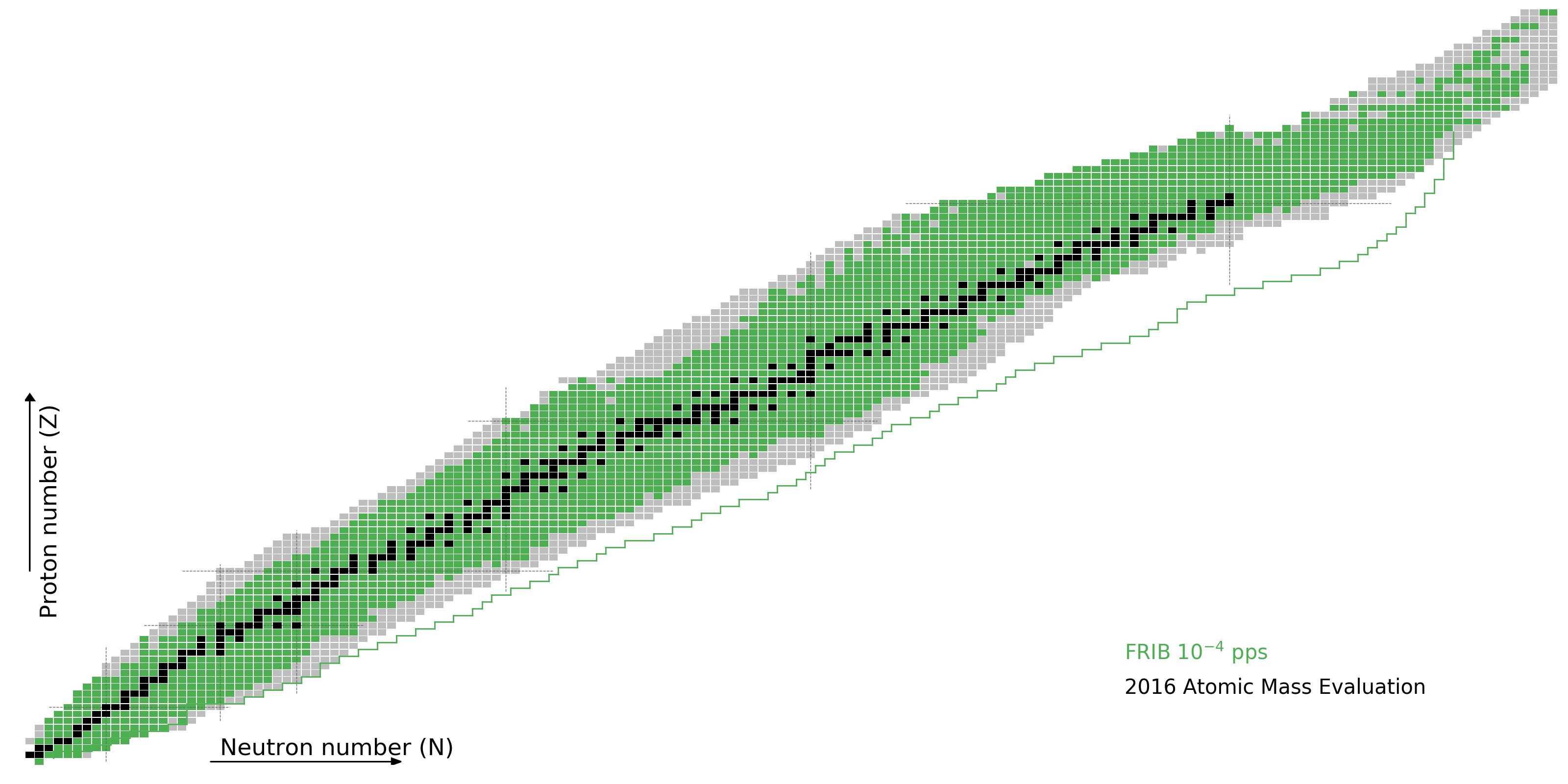## What do we know?

Neutron capture rates## What do we know?

As of today, to varying degrees of accuracy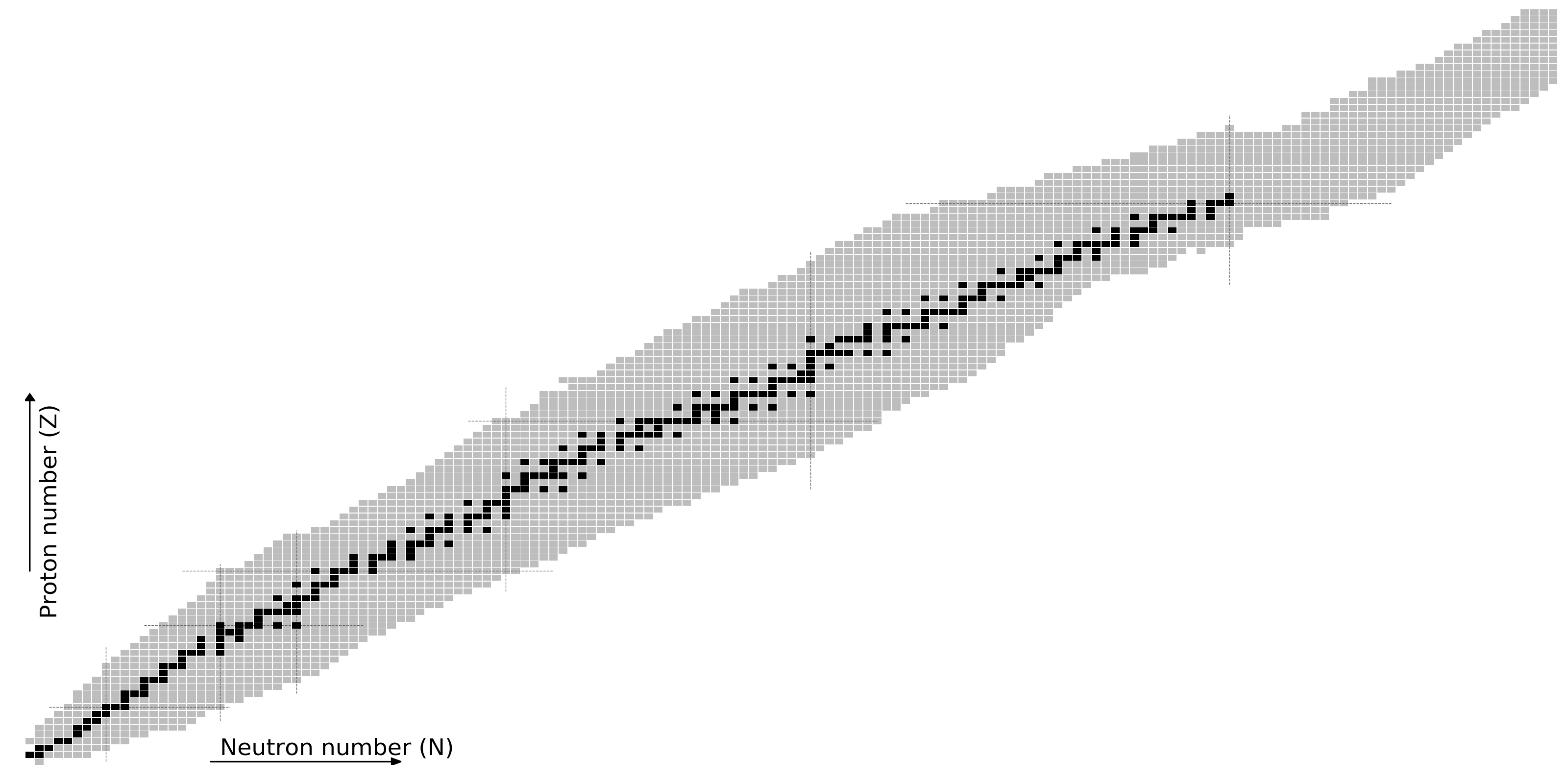## The Future

FRIB as the $r$-process machine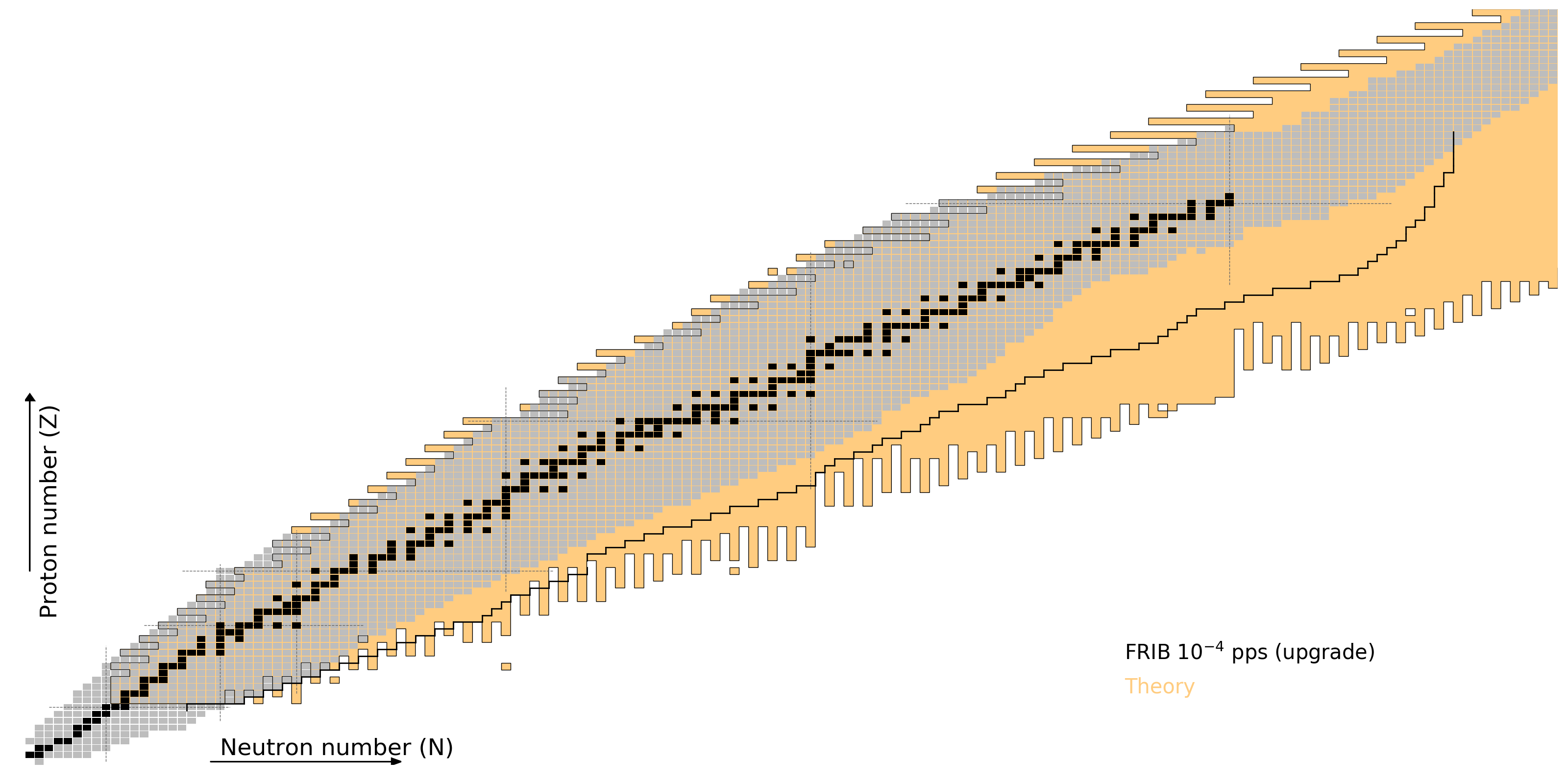## Schematic of nuclear fissionHeavy nucleus is unstable (naturally or via particle absorption) splitting into two lighter fragments

Breaking configuration is known as scission

Ensemble of events produces a fission yield

The high amount of energy released makes it interesting for observations

## How does a nucleosynthesis calculation work?combine nuclear physics inputs with astrophysical conditions

## Summary

Nuclear physics is intimately connected to astrophysics

Nucleosynthesis is one aspect of this connection

There are many different nucleosynthesis processes

Big Bang$s$-process$r$-process

The formation of the heaviest elements still remains an unsolved problem

FRIB and other facilities will help in this endeavor by constraining nuclear theories used in calculations